Index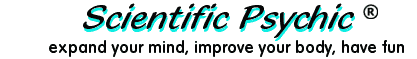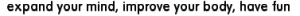The Bacteriologist Problem - part 2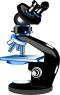A scientist is experimenting with bacteria that are one micron in diameter and that reproduce by dividing every minute into two bacteria. At 12:00 PM, she puts a single organism in a container. At precisely 1:00 PM, the container is full.

How big was the container?

The number of bacteria in the container is 2n where n is the number of minutes elapsed. The full container has 260 (two to the sixtieth power), or approximately 1.153×1018 bacteria. Since one micron (one millionth of a meter) is 1×10-4 centimeters, each bacterium occupies a volume of about 1×10-12 cubic centimeters. Multiplying times the number of organisms, we conclude that the container had a capacity of 1.153×106 cc or 1,153 liters. The container was slightly bigger than one cubic meter.

260 = 1,152,921,504,606,846,976Reader Greg Holmes submitted the following comment:

Your estimation of the volume occupied by the bacteria is not quite correct. You state that each bacterium occupies a volume of 1×10-12 cubic centimeters, or (1 micron)3. This would imply that the bacteria are cubic. However the problem states that the bacteria are spherical (1 micron in diameter). The more accurate volume occupied by each bacterium is 0.5236×10-12 cubic centimeters (4/3 × pi × r3). Further complicating the problem is the fact that spheres stacked upon one another leave a considerable amount of empty space between them. It is well documented that [in a close-packed arrangement] the ratio of volume occupied by the spheres to the total volume of 3-dimensional space is 0.7405. Therefore, the container capacity would be more precisely calculated as 0.8152×106 cc or 815.2 liters.

Volume = (number of bacteria)*(volume of each spherical bacterium)/0.7405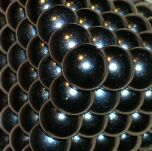Close-packed
arrangement

Strictly speaking, the problem does not state that the bacteria are "spherical". The word "diameter" is defined by the Merriam-Webster Dictionary as 1) a chord passing through the center of a figure or body, 2) the length of a straight line through the center of an object. The definition does not say that the object has to be circular or spherical. However, other sources define "diameter" as a straight line segment passing through the center of a figure, especially of a circle or sphere, and terminating at the periphery. So there you have it. The volume of the container is 1,153 liters for cubic bacteria, or 815 liters for round bacteria arranged in a close-packed arrangement.

Carl Friedrich Gauss proved that the greatest fraction of space occupied by spheres in a regular lattice arrangement is: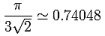Reader Alfred W. said:

The number of bacteria is actually 1 + 259, not 260. Remember that you are starting with a single bacterium. In the first minute the one becomes two. This clearly affects the container size calculation....

Actually, the answer given above is correct. The single bacterium that is placed in the dish at 12:00 PM will become two bacteria at 12:01 PM, this corresponds to the change from 20 to 21. From 12:01 PM to 12:02 PM the number of bacteria increases from 21 to 22. By continuing with this logic for each minute, the bacteria present at 12:59 PM will double at 1:00 PM going from 259 to 260 which is the number of organisms on which the volume is computed.